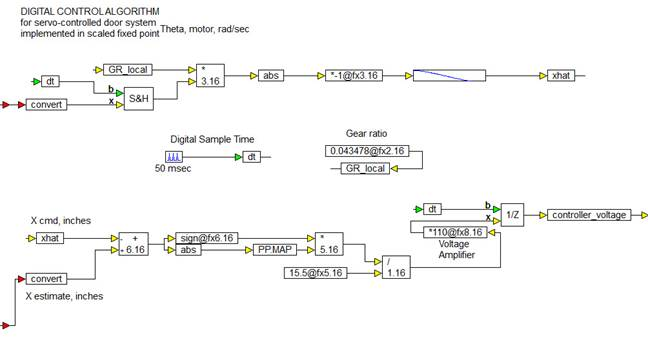##### Completing the controller implementation

To complete the controller implementation, the control law segment is connected to the Encoder feedback segment, and the output of the unitDelay block to the output:The two inputs to the fixed-point Controller are scaled to the correct data types using convert blocks. The convert block connected to the encoder feedback needs a radix point precision of at least 8 bits while the convert block connected to the command input can be 6 bits.

When this simulation is executed, the controller is implemented in 16-bit scaled precision, while the rest of the simulation runs in double precision floating-point. This lets you simulate and validate the performance of the controller, as it would execute on the fixed-point target.